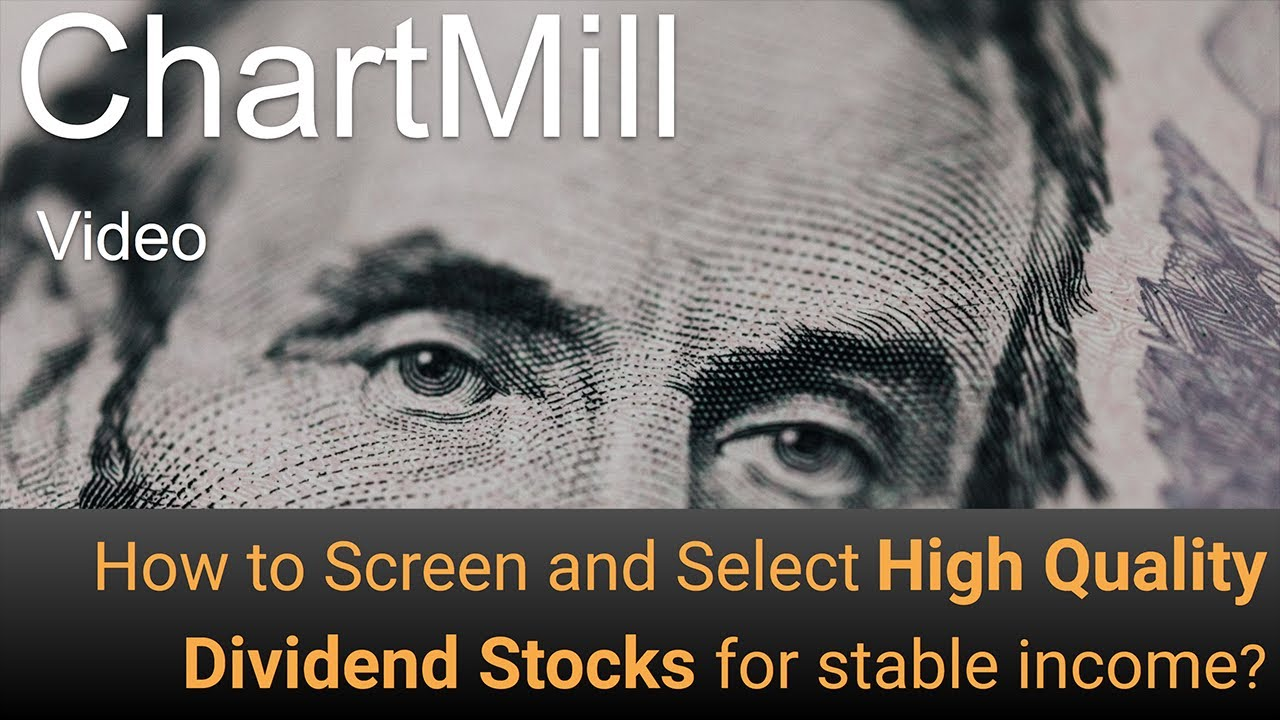# Fundamental Dividend Filters

In this article we will discuss the fundamental filters related to the Dividendof a stock. All these filters are available in our stock screener and can be found on the Fundamental tab.

## Dividend Yield

Dividend is the portion of the final profit paid to the shareholders. But what gross return is achieved with the dividend? The dividend yield is calculated by dividing the total annual dividend by the current share price. For example, if you only want shares with a dividend yield greater than 4%, you can use this filter.

## Dividend Growth

The payment of a dividend is just one thing. Yet, there is another important dividend filter which you should definitely keep in mind. The dividend growth filter tells you exactly how much the dividend yield has risen over the last 5 years. High dividend shares will be less likely to increase their dividend than shares that start with a lower dividend policy but consistently increase their dividend yield year after year.

An example: you buy a share of 20 dollars and receive a dividend yield of 5%, which amounts to 1 dollar. Suppose the return over the next five years has increased by 20%, giving you 1,20 instead of 1 dollar. This means an increase of the dividend of 6% compared to your original purchase price. Suppose you make the same investment in another 20 dollar share but you initially received 4 instead of 5% dividend (0.8 dollar). However, the dividend yield in this share has risen by 75% after five years. This means that your dividend has risen to 1.4 dollars and the dividend yield at the original purchase price is no longer 5% but 7%.

## Dividend Payout

How much of the net profit does the company distribute to the shareholders? To find out, the payout filter was included. The payout is calculated by dividing the dividend by the net profit of the company (after taxes). The result is then multiplied by 100% to calculate the ratio. New companies generally know a low payout ratio because a large part of the profit is reinvested (with the intention of realizing / financing further growth). Large-scale companies, where the further opportunities for growth are a lot smaller, usually outweigh a greater part of the profit in the form of a dividend, and will also do every effort to keep the dividend as stable as possible.

### Related Articles### Related Screens### Related videos